# How to use the FIND function in Excel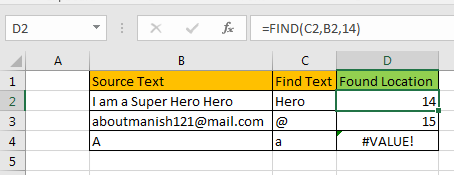In this article, we will learn How to use the FIND function in Excel.

Find any text in Excel

In Excel, you need to find the position of a text. Either you can count manually watching closely each cell value. Excel doesn't want you to do that and neither do we. For example finding the position of space character to divide values wherever space exists like separating first name and last name from the full name. So let's see the FIND function syntax and an example to illustrate its use.

In the  cell Jimmy Kimmel, Space character comes right after the y and before K. 6th is the occurrence of Space char in word starting from J.

FIND Function in Excel

FIND function just needs two arguments (third optional). Given a partial text or single character to find within a text value is done using the FIND function.

FIND function Syntax:

 =FIND(find_text, within_text, [start_num])

Find_text : The character or set of characters you want to find in string.

Within_text : The text or cell reference in which you want to find the text

[start_num] : optional. The starting point of search.

Example :

All of these might be confusing to understand. Let's understand how to use the function using an example. Here Just to explain FIND function better, I have prepared this data.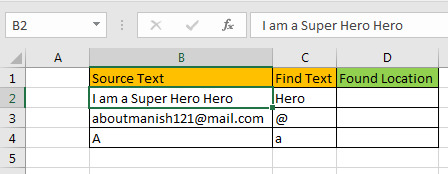In Cell D2 to D4, I want to find the location of “Hero” in cell B2, “@” in cell B3 and “a” in B4, respectively.

I write this FIND formula in Cell D2 and drag it down to cell D4.

Use the Formula in D4 cell

 =FIND(C4,B4,14)

And now i have the location of the given text: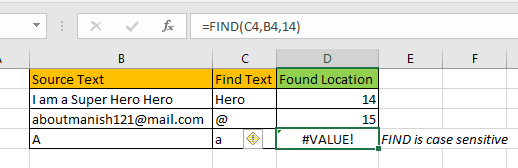Find the Second, Third and Nth Occurrence of Given Characters in the Strings.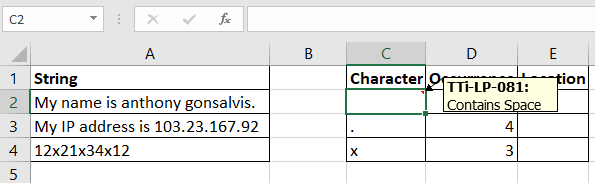Here we have some strings in range A2:A4. In cell C2, C3, and C4 we have mentioned the characters that we want to search in the strings. In D2, D3, and D4 we have mentioned the occurrence of the character. In the adjacent cell I want to get the position of these occurrences of the characters.

Write this formula in the cell E2 and drag down.

 =FIND("~", SUBSTITUTE(A2,C2,"~",D2))

This returns the exact positions (19) of the mentioned occurrence (4) of the space character in the string.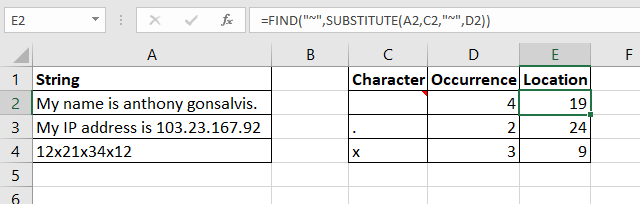How does it work?

The technique is quite simple. As we know the SUBSTITUTE function of Excel replaces the given occurrence of a text in string with the given text. We use this property.

So the formula works from inside.

SUBSTITUTE(A2,C2,"~",D2): This part resolves to SUBSTITUTE("My name is anthony gonsalvis."
," ","~",4). Which ultimately gives us the string "My name is anthony~gonsalvis."

P.S. : the fourth occurrence of space is replaced with "~". I replaced space with "~" because I am sure that this character will not appear in the string by default. You can use any character that you are sure will not appear in the string. You can use the CHAR function to insert symbols.

Search substring in Excel

Here we have two columns. Substring in Column B and Given string in Column A.

Write the formula in the C2 cell.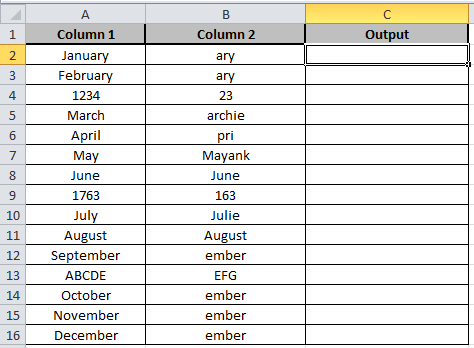Formula:

 =ISNUMBER(FIND(B2,A2))

Explanation:

Find function takes the substring from the B2 cell of Column B and it then matches it with the given string in the A2 cell of Column A.

ISNUMBER checks if the string matches, it returns True else it returns False.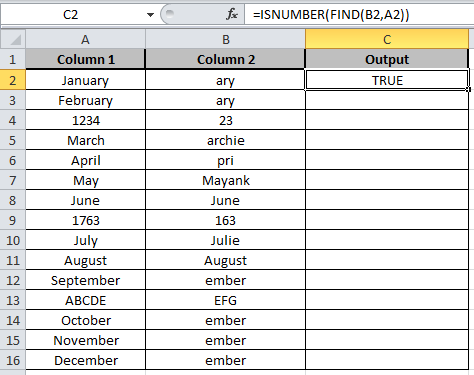Copy the formula in other cells, select the cells taking the first cell where the formula is already applied, use shortcut key Ctrl + D.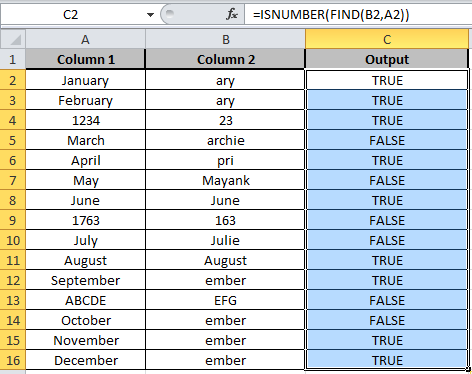As you can see the output in column C shows True and False representing whether substring is there or not.

Here are all the observational notes using the FIND function in Excel
Notes :

FIND returns the first found location by default.

If you want a second found location of text then provide start_num and it should be greater than first location of the text.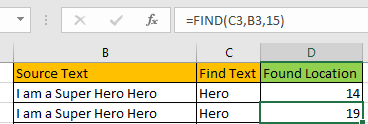If the given text is not found, FIND formula will return #VALUE error.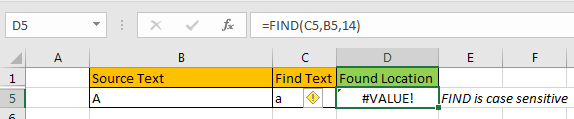FIND is case sensitive. If you need an case insentive function, use the SEARCH function.FIND does not support wildcard characters. Use the SEARCH function if you need to use wildcards to find text in a string.

Hope this article about How to use the FIND function in Excel is explanatory. Find more articles on searching partial text and related Excel formulas here. If you liked our blogs, share it with your friends on Facebook. And also you can follow us on Twitter and Facebook. We would love to hear from you, do let us know how we can improve, complement or innovate our work and make it better for you. Write to us at info@exceltip.com.

Related Articles :

How to use the SEARCH FUNCTION : It works same as the FIND function with only one difference of finding case insensitive ( like a or A are considered the same) in Excel.

Searching a String for a Specific Substring in Excel : Find cells if cell contains given word in Excel using the FIND or SEARCH function.

Highlight cells that contain specific text : Highlight cells if cell contains given word in Excel using the formula under Conditional formatting

How to Check if a string contains one of many texts in Excel : lookup cells if cell contains from given multiple words in Excel using the FIND or SEARCH function.

Count Cells that contain specific text : Count number of cells if cell contains given text using one formula in Excel.

How to lookup cells having certain text and returns the Certain Text in Excel : find cells if cell contains certain text and returns required results using the IF function in Excel.

Popular Articles :

How to use the IF Function in Excel : The IF statement in Excel checks the condition and returns a specific value if the condition is TRUE or returns another specific value if FALSE.

How to use the VLOOKUP Function in Excel : This is one of the most used and popular functions of excel that is used to lookup value from different ranges and sheets.

How to use the SUMIF Function in Excel : This is another dashboard essential function. This helps you sum up values on specific conditions.

How to use the COUNTIF Function in Excel : Count values with conditions using this amazing function. You don't need to filter your data to count specific values. Countif function is essential to prepare your dashboard.

Terms and Conditions of use

The applications/code on this site are distributed as is and without warranties or liability. In no event shall the owner of the copyrights, or the authors of the applications/code be liable for any loss of profit, any problems or any damage resulting from the use or evaluation of the applications/code.